$$\require{cancel}$$

7.E: Work and Kinetic Energy (Exercises)

•• Contributed by OpenStax
• General Physics at OpenStax CNX

Conceptual Questions

7.1 Work

1. Give an example of something we think of as work in everyday circumstances that is not work in the scientific sense. Is energy transferred or changed in form in your example? If so, explain how this is accomplished without doing work.
2. Give an example of a situation in which there is a force and a displacement, but the force does no work. Explain why it does no work.
3. Describe a situation in which a force is exerted for a long time but does no work. Explain.
4. A body moves in a circle at constant speed. Does the centripetal force that accelerates the body do any work? Explain.
5. Suppose you throw a ball upward and catch it when it returns at the same height. How much work does the gravitational force do on the ball over its entire trip?
6. Why is it more difficult to do sit-ups while on a slant board than on a horizontal surface? (See below.)1. As a young man, Tarzan climbed up a vine to reach his tree house. As he got older, he decided to build and use a staircase instead. Since the work of the gravitational force mg is path independent, what did the King of the Apes gain in using stairs?

7.2 Kinetic Energy

1. A particle of m has a velocity of vx $$\hat{i}$$ + vy $$\hat{j}$$ + vz $$\hat{k}$$. Is its kinetic energy given by m(vx2 $$\hat{i}$$ + vy2 $$\hat{j}$$ + vz2 $$\hat{k}$$)/2? If not, what is the correct expression?
2. One particle has mass m and a second particle has mass 2m. The second particle is moving with speed v and the first with speed 2v. How do their kinetic energies compare?
3. A person drops a pebble of mass m1 from a height h, and it hits the floor with kinetic energy K. The person drops another pebble of mass m2 from a height of 2h, and it hits the floor with the same kinetic energy K. How do the masses of the pebbles compare?

7.3 Work-Energy Theorem

11. Under what conditions would it lose energy?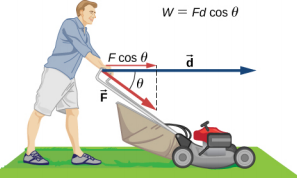1. Work done on a system puts energy into it. Work done by a system removes energy from it. Give an example for each statement.
2. Two marbles of masses m and 2m are dropped from a height h. Compare their kinetic energies when they reach the ground.
3. Compare the work required to accelerate a car of mass 2000 kg from 30.0 to 40.0 km/h with that required for an acceleration from 50.0 to 60.0 km/h.
4. Suppose you are jogging at constant velocity. Are you doing any work on the environment and vice versa?
5. Two forces act to double the speed of a particle, initially moving with kinetic energy of 1 J. One of the forces does 4 J of work. How much work does the other force do?

7.4 Power

1. Most electrical appliances are rated in watts. Does this rating depend on how long the appliance is on? (When off, it is a zero-watt device.) Explain in terms of the definition of power.
2. Explain, in terms of the definition of power, why energy consumption is sometimes listed in kilowatt-hours rather than joules. What is the relationship between these two energy units?
3. A spark of static electricity, such as that you might receive from a doorknob on a cold dry day, may carry a few hundred watts of power. Explain why you are not injured by such a spark.
4. Does the work done in lifting an object depend on how fast it is lifted? Does the power expended depend on how fast it is lifted?
5. Can the power expended by a force be negative?
6. How can a 50-W light bulb use more energy than a 1000-W oven?

Problems

7.1 Work

1. How much work does a supermarket checkout attendant do on a can of soup he pushes 0.600 m horizontally with a force of 5.00 N?
2. A 75.0-kg person climbs stairs, gaining 2.50 m in height. Find the work done to accomplish this task.
3. (a) Calculate the work done on a 1500-kg elevator car by its cable to lift it 40.0 m at constant speed, assuming friction averages 100 N. (b) What is the work done on the lift by the gravitational force in this process? (c) What is the total work done on the lift?
4. Suppose a car travels 108 km at a speed of 30.0 m/s, and uses 2.0 gal of gasoline. Only 30% of the gasoline goes into useful work by the force that keeps the car moving at constant speed despite friction. (The energy content of gasoline is about 140 MJ/gal.) (a) What is the magnitude of the force exerted to keep the car moving at constant speed? (b) If the required force is directly proportional to speed, how many gallons will be used to drive 108 km at a speed of 28.0 m/s?
5. Calculate the work done by an 85.0-kg man who pushes a crate 4.00 m up along a ramp that makes an angle of 20.0° with the horizontal (see below). He exerts a force of 500 N on the crate parallel to the ramp and moves at a constant speed. Be certain to include the work he does on the crate and on his body to get up the ramp.1. How much work is done by the boy pulling his sister 30.0 m in a wagon as shown below? Assume no friction acts on the wagon.1. A shopper pushes a grocery cart 20.0 m at constant speed on level ground, against a 35.0 N frictional force. He pushes in a direction 25.0° below the horizontal. (a) What is the work done on the cart by friction? (b) What is the work done on the cart by the gravitational force? (c) What is the work done on the cart by the shopper? (d) Find the force the shopper exerts, using energy considerations. (e) What is the total work done on the cart?
2. Suppose the ski patrol lowers a rescue sled and victim, having a total mass of 90.0 kg, down a 60.0° slope at constant speed, as shown below. The coefficient of friction between the sled and the snow is 0.100. (a) How much work is done by friction as the sled moves 30.0 m along the hill? (b) How much work is done by the rope on the sled in this distance? (c) What is the work done by the gravitational force on the sled? (d) What is the total work done?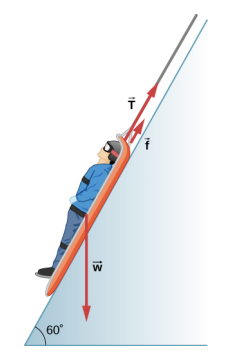1. A constant 20-N force pushes a small ball in the direction of the force over a distance of 5.0 m. What is the work done by the force?
2. A toy cart is pulled a distance of 6.0 m in a straight line across the floor. The force pulling the cart has a magnitude of 20 N and is directed at 37° above the horizontal. What is the work done by this force?
3. A 5.0-kg box rests on a horizontal surface. The coefficient of kinetic friction between the box and surface is $$\mu_{K}$$ = 0.50. A horizontal force pulls the box at constant velocity for 10 cm. Find the work done by (a) the applied horizontal force, (b) the frictional force, and (c) the net force.
4. A sled plus passenger with total mass 50 kg is pulled 20 m across the snow (µk = 0.20) at constant velocity by a force directed 25° above the horizontal. Calculate (a) the work of the applied force, (b) the work of friction, and (c) the total work.
5. Suppose that the sled plus passenger of the preceding problem is pushed 20 m across the snow at constant velocity by a force directed 30° below the horizontal. Calculate (a) the work of the applied force, (b) the work of friction, and (c) the total work.
6. How much work does the force F(x) = (−2.0/x) N do on a particle as it moves from x = 2.0 m to x = 5.0 m?
7. How much work is done against the gravitational force on a 5.0-kg briefcase when it is carried from the ground floor to the roof of the Empire State Building, a vertical climb of 380 m?
8. It takes 500 J of work to compress a spring 10 cm. What is the force constant of the spring?
9. A bungee cord is essentially a very long rubber band that can stretch up to four times its unstretched length. However, its spring constant varies over its stretch [see Menz, P.G. “The Physics of Bungee Jumping.” The Physics Teacher (November 1993) 31: 483-487]. Take the length of the cord to be along the x-direction and define the stretch x as the length of the cord l minus its un-stretched length l0 ; that is, x = l − l0 (see below). Suppose a particular bungee cord has a spring constant, for 0 ≤ x ≤ 4.88 m, of k1 = 204 N/m and for 4.88 m ≤ x, of k2 = 111 N/m. (Recall that the spring constant is the slope of the force F(x) versus its stretch x.) (a) What is the tension in the cord when the stretch is 16.7 m (the maximum desired for a given jump)? (b) How much work must be done against the elastic force of the bungee cord to stretch it 16.7 m?Figure 7.16 - (credit: Graeme Churchard)
1. A bungee cord exerts a nonlinear elastic force of magnitude F(x) = k1x + k2x3, where x is the distance the cord is stretched, k1 = 204 N/m and k2 = −0.233 N/m3. How much work must be done on the cord to stretch it 16.7 m?
2. Engineers desire to model the magnitude of the elastic force of a bungee cord using the equation $$F(x) = a \Bigg[\frac{x + 9\; m}{9\; m} − \left(\dfrac{9\; m}{x + 9\; m}\right)^{2} \Bigg],$$where x is the stretch of the cord along its length and a is a constant. If it takes 22.0 kJ of work to stretch the cord by 16.7 m, determine the value of the constant a.
3. A particle moving in the xy-plane is subject to a force $$\vec{F}(x, y) = (50\; N \; \cdotp m^{2}) \frac{(x \hat{i} + y \hat{j})}{(x^{2} + y^{2})^{3/2}},$$where x and y are in meters. Calculate the work done on the particle by this force, as it moves in a straight line from the point (3 m, 4 m) to the point (8 m, 6 m).
4. A particle moves along a curved path y(x) = (10 m){1 + cos[(0.1 m−1)x]}, from x = 0 to x = 10$$\pi$$ m, subject to a tangential force of variable magnitude F(x) = (10 N)sin[(0.1 m−1)x]. How much work does the force do? (Hint: Consult a table of integrals or use a numerical integration program.)

7.2 Kinetic Energy

1. Compare the kinetic energy of a 20,000-kg truck moving at 110 km/h with that of an 80.0-kg astronaut in orbit moving at 27,500 km/h.
2. (a) How fast must a 3000-kg elephant move to have the same kinetic energy as a 65.0-kg sprinter running at 10.0 m/s? (b) Discuss how the larger energies needed for the movement of larger animals would relate to metabolic rates.
3. Estimate the kinetic energy of a 90,000-ton aircraft carrier moving at a speed of at 30 knots. You will need to look up the definition of a nautical mile to use in converting the unit for speed, where 1 knot equals 1 nautical mile per hour.
4. Calculate the kinetic energies of (a) a 2000.0-kg automobile moving at 100.0 km/h; (b) an 80.-kg runner sprinting at 10. m/s; and (c) a 9.1 x 10−31-kg electron moving at 2.0 x 107 m/s.
5. A 5.0-kg body has three times the kinetic energy of an 8.0-kg body. Calculate the ratio of the speeds of these bodies.
6. An 8.0-g bullet has a speed of 800 m/s. (a) What is its kinetic energy? (b) What is its kinetic energy if the speed is halved?

7.3 Work-Energy Theorem

1. (a) Calculate the force needed to bring a 950-kg car to rest from a speed of 90.0 km/h in a distance of 120 m (a fairly typical distance for a non-panic stop). (b) Suppose instead the car hits a concrete abutment at full speed and is brought to a stop in 2.00 m. Calculate the force exerted on the car and compare it with the force found in part (a).
2. A car’s bumper is designed to withstand a 4.0-km/h (1.1-m/s) collision with an immovable object without damage to the body of the car. The bumper cushions the shock by absorbing the force over a distance. Calculate the magnitude of the average force on a bumper that collapses 0.200 m while bringing a 900-kg car to rest from an initial speed of 1.1 m/s.
3. Boxing gloves are padded to lessen the force of a blow. (a) Calculate the force exerted by a boxing glove on an opponent’s face, if the glove and face compress 7.50 cm during a blow in which the 7.00-kg arm and glove are brought to rest from an initial speed of 10.0 m/s. (b) Calculate the force exerted by an identical blow in the gory old days when no gloves were used, and the knuckles and face would compress only 2.00 cm. Assume the change in mass by removing the glove is negligible. (c) Discuss the magnitude of the force with glove on. Does it seem high enough to cause damage even though it is lower than the force with no glove?
4. Using energy considerations, calculate the average force a 60.0-kg sprinter exerts backward on the track to accelerate from 2.00 to 8.00 m/s in a distance of 25.0 m, if he encounters a headwind that exerts an average force of 30.0 N against him.
5. A 5.0-kg box has an acceleration of 2.0 m/s2 when it is pulled by a horizontal force across a surface with $$\mu_{K}$$ = 0.50. Find the work done over a distance of 10 cm by (a) the horizontal force, (b) the frictional force, and (c) the net force. (d) What is the change in kinetic energy of the box?
6. A constant 10-N horizontal force is applied to a 20-kg cart at rest on a level floor. If friction is negligible, what is the speed of the cart when it has been pushed 8.0 m?
7. In the preceding problem, the 10-N force is applied at an angle of 45° below the horizontal. What is the speed of the cart when it has been pushed 8.0 m?
8. Compare the work required to stop a 100-kg crate sliding at 1.0 m/s and an 8.0-g bullet traveling at 500 m/s.
9. A wagon with its passenger sits at the top of a hill. The wagon is given a slight push and rolls 100 m down a 10° incline to the bottom of the hill. What is the wagon’s speed when it reaches the end of the incline. Assume that the retarding force of friction is negligible.
10. An 8.0-g bullet with a speed of 800 m/s is shot into a wooden block and penetrates 20 cm before stopping. What is the average force of the wood on the bullet? Assume the block does not move.
11. A 2.0-kg block starts with a speed of 10 m/s at the bottom of a plane inclined at 37° to the horizontal. The coefficient of sliding friction between the block and plane is $$mu_{k}$$ = 0.30. (a) Use the work-energy principle to determine how far the block slides along the plane before momentarily coming to rest. (b) After stopping, the block slides back down the plane. What is its speed when it reaches the bottom? (Hint: For the round trip, only the force of friction does work on the block.)
12. When a 3.0-kg block is pushed against a massless spring of force constant constant 4.5 x 103 N/m, the spring is compressed 8.0 cm. The block is released, and it slides 2.0 m (from the point at which it is released) across a horizontal surface before friction stops it. What is the coefficient of kinetic friction between the block and the surface?
13. A small block of mass 200 g starts at rest at A, slides to B where its speed is vB = 8.0 m/s, then slides along the horizontal surface a distance 10 m before coming to rest at C. (See below.) (a) What is the work of friction along the curved surface? (b) What is the coefficient of kinetic friction along the horizontal surface?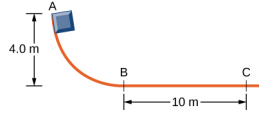1. A small object is placed at the top of an incline that is essentially frictionless. The object slides down the incline onto a rough horizontal surface, where it stops in 5.0 s after traveling 60 m. (a) What is the speed of the object at the bottom of the incline and its acceleration along the horizontal surface? (b) What is the height of the incline?
2. When released, a 100-g block slides down the path shown below, reaching the bottom with a speed of 4.0 m/s. How much work does the force of friction do?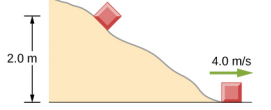1. A 0.22LR-caliber bullet like that mentioned in Example 7.10 is fired into a door made of a single thickness of 1-inch pine boards. How fast would the bullet be traveling after it penetrated through the door?
2. A sled starts from rest at the top of a snow-covered incline that makes a 22° angle with the horizontal. After sliding 75 m down the slope, its speed is 14 m/s. Use the work-energy theorem to calculate the coefficient of kinetic friction between the runners of the sled and the snowy surface.

7.4 Power

1. A person in good physical condition can put out 100 W of useful power for several hours at a stretch, perhaps by pedaling a mechanism that drives an electric generator. Neglecting any problems of generator efficiency and practical considerations such as resting time: (a) How many people would it take to run a 4.00-kW electric clothes dryer? (b) How many people would it take to replace a large electric power plant that generates 800 MW?
2. What is the cost of operating a 3.00-W electric clock for a year if the cost of electricity is $0.0900 per kW • h? 3. A large household air conditioner may consume 15.0 kW of power. What is the cost of operating this air conditioner 3.00 h per day for 30.0 d if the cost of electricity is$0.110 per kW • h?
4. (a) What is the average power consumption in watts of an appliance that uses 5.00 kW • h of energy per day? (b) How many joules of energy does this appliance consume in a year?
5. (a) What is the average useful power output of a person who does 6.00 x 106 J of useful work in 8.00 h? (b) Working at this rate, how long will it take this person to lift 2000 kg of bricks 1.50 m to a platform? (Work done to lift his body can be omitted because it is not considered useful output here.)
6. A 500-kg dragster accelerates from rest to a final speed of 110 m/s in 400 m (about a quarter of a mile) and encounters an average frictional force of 1200 N. What is its average power output in watts and horsepower if this takes 7.30 s?
7. (a) How long will it take an 850-kg car with a useful power output of 40.0 hp (1 hp equals 746 W) to reach a speed of 15.0 m/s, neglecting friction? (b) How long will this acceleration take if the car also climbs a 3.00-m high hill in the process?
8. (a) Find the useful power output of an elevator motor that lifts a 2500-kg load a height of 35.0 m in 12.0 s, if it also increases the speed from rest to 4.00 m/s. Note that the total mass of the counterbalanced system is 10,000 kg—so that only 2500 kg is raised in height, but the full 10,000 kg is accelerated. (b) What does it cost, if electricity is \$0.0900 per kW • h ?
9. (a) How long would it take a 1.50 x 105-kg airplane with engines that produce 100 MW of power to reach a speed of 250 m/s and an altitude of 12.0 km if air resistance were negligible? (b) If it actually takes 900 s, what is the power? (c) Given this power, what is the average force of air resistance if the airplane takes 1200 s? (Hint: You must find the distance the plane travels in 1200 s assuming constant acceleration.)
10. Calculate the power output needed for a 950-kg car to climb a 2.00° slope at a constant 30.0 m/s while encountering wind resistance and friction totaling 600 N.
11. A man of mass 80 kg runs up a flight of stairs 20 m high in 10 s. (a) how much power is used to lift the man? (b) If the man’s body is 25% efficient, how much power does he expend?
12. The man of the preceding problem consumes approximately 1.05 x 107 J (2500 food calories) of energy per day in maintaining a constant weight. What is the average power he produces over a day? Compare this with his power production when he runs up the stairs.
13. An electron in a television tube is accelerated uniformly from rest to a speed of 8.4 x 107 m/s over a distance of 2.5 cm. What is the power delivered to the electron at the instant that its displacement is 1.0 cm?
14. Coal is lifted out of a mine a vertical distance of 50 m by an engine that supplies 500 W to a conveyer belt. How much coal per minute can be brought to the surface? Ignore the effects of friction.
15. A girl pulls her 15-kg wagon along a flat sidewalk by applying a 10-N force at 37° to the horizontal. Assume that friction is negligible and that the wagon starts from rest. (a) How much work does the girl do on the wagon in the first 2.0 s. (b) How much instantaneous power does she exert at t = 2.0 s ?
16. A typical automobile engine has an efficiency of 25%. Suppose that the engine of a 1000-kg automobile has a maximum power output of 140 hp. What is the maximum grade that the automobile can climb at 50 km/h if the frictional retarding force on it is 300 N?
17. When jogging at 13 km/h on a level surface, a 70-kg man uses energy at a rate of approximately 850 W. Using the facts that the “human engine” is approximately 25% efficient, determine the rate at which this man uses energy when jogging up a 5.0° slope at this same speed. Assume that the frictional retarding force is the same in both cases.

1. A cart is pulled a distance D on a flat, horizontal surface by a constant force F that acts at an angle $$\theta$$ with the horizontal direction. The other forces on the object during this time are gravity (Fw), normal forces (FN1) and (FN2), and rolling frictions Fr1 and Fr2, as shown below. What is the work done by each force?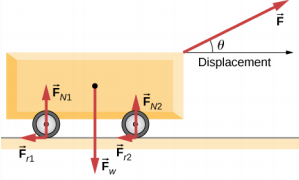1. Consider a particle on which several forces act, one of which is known to be constant in time: $$\vec{F}_{1}$$ = (3 N) $$\hat{i}$$ + (4 N) $$\hat{j}$$. As a result, the particle moves along the x-axis from x = 0 to x = 5 m in some time interval. What is the work done by $$\vec{F}_{1}$$?
2. Consider a particle on which several forces act, one of which is known to be constant in time: $$\vec{F}_{1}$$ = (3 N) $$\hat{i}$$ + (4 N) $$\hat{j}$$. As a result, the particle moves first along the x-axis from x = 0 to x = 5 m and then parallel to the y-axis from y = 0 to y = 6 m. What is the work done by $$\vec{F}_{1}$$?
3. Consider a particle on which several forces act, one of which is known to be constant in time: $$\vec{F}_{1}$$ = (3 N) $$\hat{i}$$ + (4 N) $$\hat{j}$$. As a result, the particle moves along a straight path from a Cartesian coordinate of (0 m, 0 m) to (5 m, 6 m). What is the work done by $$\vec{F}_{1}$$?
4. Consider a particle on which a force acts that depends on the position of the particle. This force is given by $$\vec{F}_{1}$$ = (2y) $$\hat{i}$$ + (3x) $$\hat{j}$$. Find the work done by this force when the particle moves from the origin to a point 5 meters to the right on the x-axis.
5. A boy pulls a 5-kg cart with a 20-N force at an angle of 30° above the horizontal for a length of time. Over this time frame, the cart moves a distance of 12 m on the horizontal floor. (a) Find the work done on the cart by the boy. (b) What will be the work done by the boy if he pulled with the same force horizontally instead of at an angle of 30° above the horizontal over the same distance?
6. A crate of mass 200 kg is to be brought from a site on the ground floor to a third floor apartment. The workers know that they can either use the elevator first, then slide it along the third floor to the apartment, or first slide the crate to another location marked C below, and then take the elevator to the third floor and slide it on the third floor a shorter distance. The trouble is that the third floor is very rough compared to the ground floor. Given that the coefficient of kinetic friction between the crate and the ground floor is 0.100 and between the crate and the third floor surface is 0.300, find the work needed by the workers for each path shown from A to E. Assume that the force the workers need to do is just enough to slide the crate at constant velocity (zero acceleration). Note: The work by the elevator against the force of gravity is not done by the workers.1. A hockey puck of mass 0.17 kg is shot across a rough floor with the roughness different at different places, which can be described by a position-dependent coefficient of kinetic friction. For a puck moving along the x-axis, the coefficient of kinetic friction is the following function of x, where x is in m: $$\mu$$(x) = 0.1 + 0.05x. Find the work done by the kinetic frictional force on the hockey puck when it has moved (a) from x = 0 to x = 2 m, and (b) from x = 2 m to x = 4 m.
2. A horizontal force of 20 N is required to keep a 5.0 kg box traveling at a constant speed up a frictionless incline for a vertical height change of 3.0 m. (a) What is the work done by gravity during this change in height? (b) What is the work done by the normal force? (c) What is the work done by the horizontal force?
3. A 7.0-kg box slides along a horizontal frictionless floor at 1.7 m/s and collides with a relatively massless spring that compresses 23 cm before the box comes to a stop. (a) How much kinetic energy does the box have before it collides with the spring? (b) Calculate the work done by the spring. (c) Determine the spring constant of the spring.
4. You are driving your car on a straight road with a coefficient of friction between the tires and the road of 0.55. A large piece of debris falls in front of your view and you immediate slam on the brakes, leaving a skid mark of 30.5 m (100-feet) long before coming to a stop. A policeman sees your car stopped on the road, looks at the skid mark, and gives you a ticket for traveling over the 13.4 m/s (30 mph) speed limit. Should you fight the speeding ticket in court?
5. A crate is being pushed across a rough floor surface. If no force is applied on the crate, the crate will slow down and come to a stop. If the crate of mass 50 kg moving at speed 8 m/s comes to rest in 10 seconds, what is the rate at which the frictional force on the crate takes energy away from the crate?
6. Suppose a horizontal force of 20 N is required to maintain a speed of 8 m/s of a 50 kg crate. (a) What is the power of this force? (b) Note that the acceleration of the crate is zero despite the fact that 20 N force acts on the crate horizontally. What happens to the energy given to the crate as a result of the work done by this 20 N force?
7. Grains from a hopper falls at a rate of 10 kg/s vertically onto a conveyor belt that is moving horizontally at a constant speed of 2 m/s. (a) What force is needed to keep the conveyor belt moving at the constant velocity? (b) What is the minimum power of the motor driving the conveyor belt?
8. A cyclist in a race must climb a 5° hill at a speed of 8 m/s. If the mass of the bike and the biker together is 80 kg, what must be the power output of the biker to achieve the goal?

Challenge Problems

1. Shown below is a 40-kg crate that is pushed at constant velocity a distance 8.0 m along a 30° incline by the horizontal force $$\vec{F}$$. The coefficient of kinetic friction between the crate and the incline is $$\mu_{k}$$ = 0.40. Calculate the work done by (a) the applied force, (b) the frictional force, (c) the gravitational force, and (d) the net force.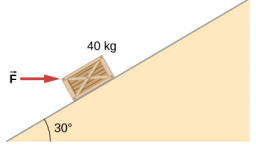1. The surface of the preceding problem is modified so that the coefficient of kinetic friction is decreased. The same horizontal force is applied to the crate, and after being pushed 8.0 m, its speed is 5.0 m/s. How much work is now done by the force of friction? Assume that the crate starts at rest.
2. The force F(x) varies with position, as shown below. Find the work done by this force on a particle as it moves from x = 1.0 m to x = 5.0 m.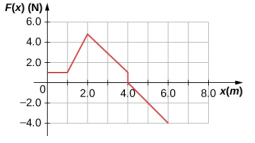1. Find the work done by the same force in Example 7.4, between the same points, A = (0, 0) and B = (2 m, 2 m) , over a circular arc of radius 2 m, centered at (0, 2 m). Evaluate the path integral using Cartesian coordinates. (Hint: You will probably need to consult a table of integrals.)
2. Answer the preceding problem using polar coordinates.
3. Find the work done by the same force in Example 7.4, between the same points, A = (0, 0) and B = (2 m, 2 m), over a circular arc of radius 2 m, centered at (2 m, 0). Evaluate the path integral using Cartesian coordinates. (Hint: You will probably need to consult a table of integrals.)
4. Answer the preceding problem using polar coordinates.
5. Constant power P is delivered to a car of mass m by its engine. Show that if air resistance can be ignored, the distance covered in a time t by the car, starting from rest, is given by s = $$\left(\frac{8P}{9m}\right)^{1/2}$$t 3/2.
6. Suppose that the air resistance a car encounters is independent of its speed. When the car travels at 15 m/ s, its engine delivers 20 hp to its wheels. (a) What is the power delivered to the wheels when the car travels at 30 m/ s? (b) How much energy does the car use in covering 10 km at 15 m/s? At 30 m/s? Assume that the engine is 25% efficient. (c) Answer the same questions if the force of air resistance is proportional to the speed of the automobile. (d) What do these results, plus your experience with gasoline consumption, tell you about air resistance?
7. Consider a linear spring, as in Figure 7.7(a), with mass M uniformly distributed along its length. The left end of the spring is fixed, but the right end, at the equilibrium position x = 0, is moving with speed v in the x-direction. What is the total kinetic energy of the spring? (Hint: First express the kinetic energy of an infinitesimal element of the spring dm in terms of the total mass, equilibrium length, speed of the right-hand end, and position along the spring; then integrate.)

Contributors

Samuel J. Ling (Truman State University), Jeff Sanny (Loyola Marymount University), and Bill Moebs with many contributing authors. This work is licensed by OpenStax University Physics under a Creative Commons Attribution License (by 4.0).